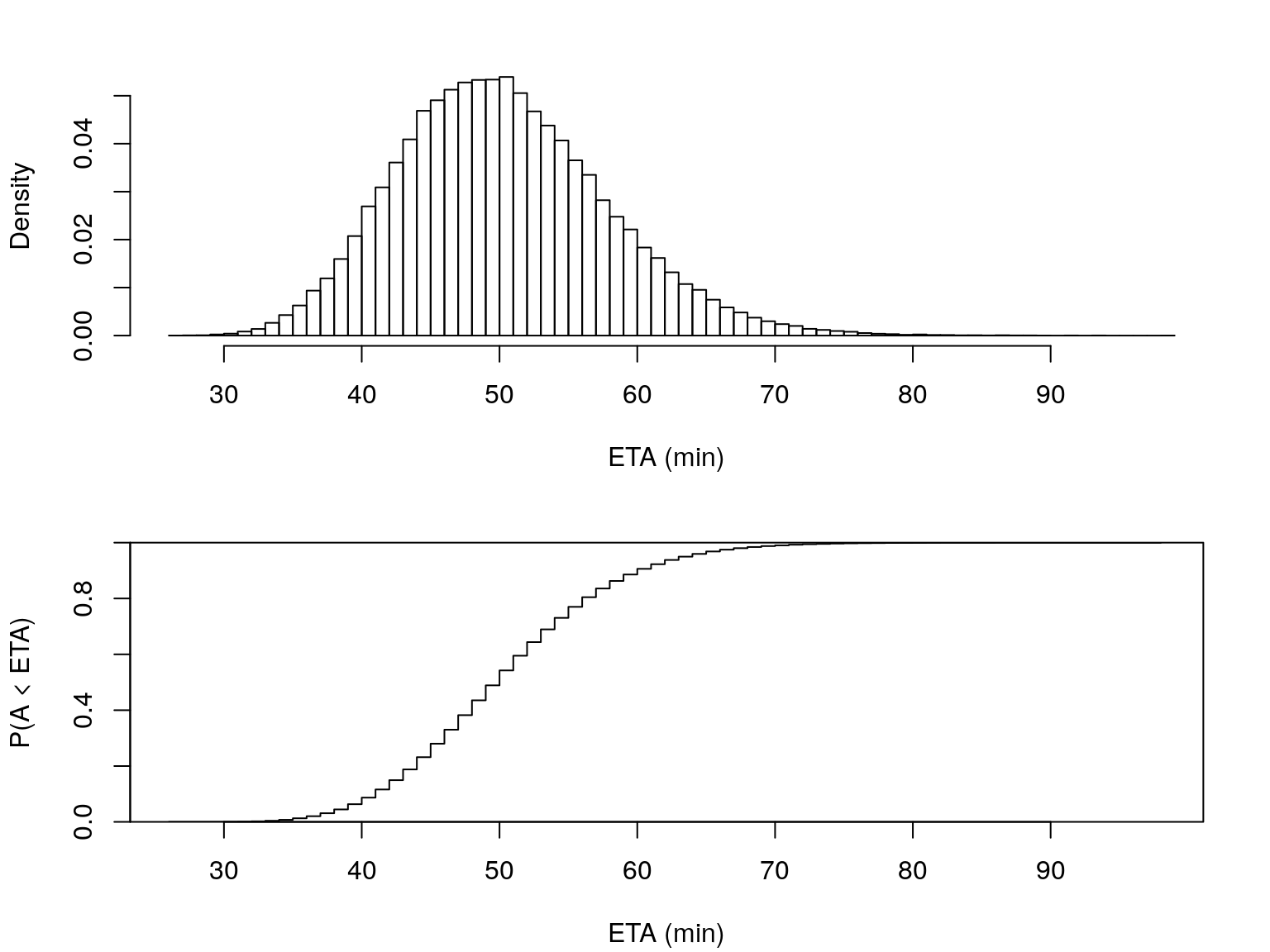# Overview

GPS positions

$$\downarrow$$

Travel times

$$\downarrow$$

Network state

$$\downarrow$$

Arrival time distributions

$$\downarrow$$

ETAs

# Part OneGPS positions$$\downarrow$$travel times

## Real-time vehicle locations

• Auckland Transport provides API for real-time data
• Vehicle locations: GPS coordinates of buses/trains
• Trip updates: arrival/departure times at bus stops
• Over 1000 buses operating at peak
• Target: ETAs updated every 30 seconds

## Vehicle state

• Unknown vehicle state $$\boldsymbol{x}_k = [x_k, \dot x_k]^\top$$, at time $$t_k$$
• $$x_k$$: distance travelled along route, meters
• $$\dot x_k$$: speed, meters per second
• Observable state $$\boldsymbol{y}_k = h(\boldsymbol{x}_k) + \boldsymbol{\epsilon}_k$$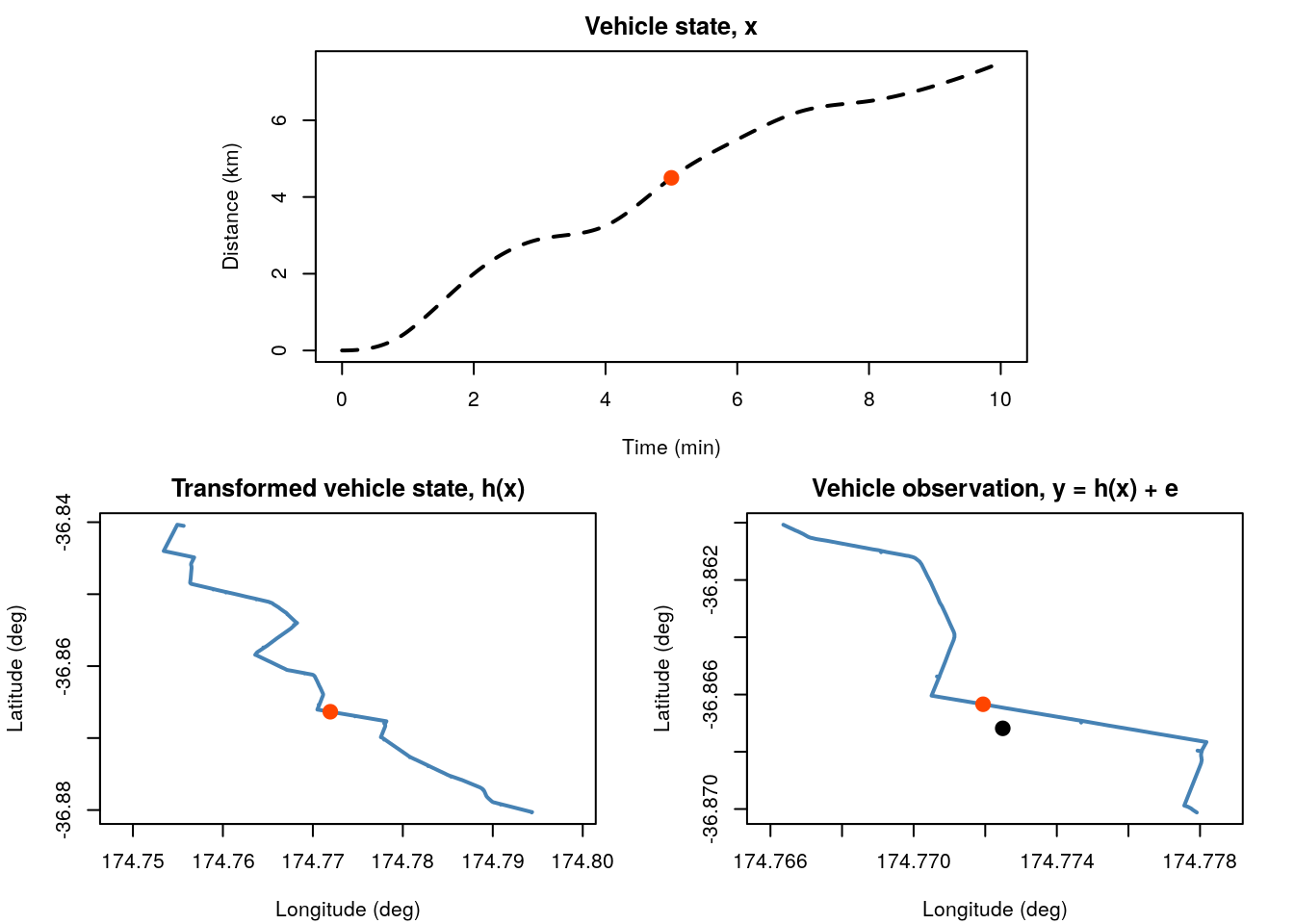## Particle filter

• Approximate vehicle state using sample of $$N$$ particles
$p(\boldsymbol{x}_{k-1} | \boldsymbol{y}_{1:k-1}) \approx \sum_{i=1}^N \boldsymbol{w}_{k-1}^{(i)} \delta_{\boldsymbol{x}_{k-1}^{(i)}}(\boldsymbol{x}_{k-1})$
• $$\boldsymbol{x}_{k-1}^{(i)}$$: state (distance, velocity) of particle $$i$$
• $$\boldsymbol{w}_{k-1}^{(i)}$$: weight of particle $$i$$
• $$\delta_{x^{(i)}}(x)$$: Dirac measure

## Particle filter ii

• Vehicle transition function, $$f$$
• Acceleration/deceleration
• Bus stop behaviour
• Traffic lights, etc.
$\boldsymbol{x}_k^{(i)} = f(\boldsymbol{x}_{k-1}^{(i)}, \sigma_x^2, ...)$
• Predicts new state before observing latest position
$p(\boldsymbol{x}_{k} | \boldsymbol{x}_{k-1}) \approx \sum_{i=1}^N \boldsymbol{w}_{k-1}^{(i)} \delta_{\boldsymbol{x}_k^{(i)}}(\boldsymbol{x}_{k})$

## Particle filter iii

• Update using likelihood function
• Distance (squared) between particle and observation
• Measurement error $$\epsilon^2$$ = GPS error (meters)
$d\left(\boldsymbol{y}_k, h(\boldsymbol{x}_k^{(i)})\right)^2 \sim Exp\left(\frac{1}{2\epsilon^2}\right)$

## Particle filter iv

• Update vehicle state by reweighting particles

$\boldsymbol{w}_k^{(i)} = \frac{\boldsymbol{w}_{k-1}^{(i)} p(\boldsymbol{y}_k | \boldsymbol{x}_k^{(i)})}{\sum_{j=1}^N \boldsymbol{w}_{k-1}^{(j)} p(\boldsymbol{y}_k | \boldsymbol{x}_k^{(j)})}$

$p(\boldsymbol{x}_k | \boldsymbol{y}_{1:k}) \approx \sum_{i=1}^N \boldsymbol{w}_{k}^{(i)} \delta_{\boldsymbol{x}_k^{(i)}}(\boldsymbol{x}_{k})$

## Estimating travel times

• Estimated once vehicle has completed each segment
• $$T_j^{(i)}$$: travel time particle $$i$$, segment $$j$$
• Computed in transition function
• Posterior estimate of travel time
$p(T_j | \boldsymbol{y}_{1:k}) \approx \sum_{i=1}^N \boldsymbol{w}_{k}^{(i)} \delta_{T_j^{(i)}}(T_{j})$

# Part TwoTravel times$$\downarrow$$Network state

• road network consists of all $$M$$ road segments
• estimate of traffic flows (as travel times)
• combines data from different routes

## Network state

• travel time (seconds) of buses along roads at time $$t_c$$
$\Theta_c = [ \theta_{1c}, \theta_{2c}, \cdots, \theta_{Mc} ]^\top$
• summarize by mean and variance
$\hat\Theta_{c|c} = \mathrm{E}(\Theta_c | T_{1:c})$ $\Sigma_{c|c} = \mathrm{Var}(\Theta_c | T_{1:c})$

## Information filter

• alternate form of Kalman filter
• replace mean and variance by
• information matrix $\mathbf{P}_{c-1|c-1} = \Sigma_{c-1|c-1}^{-1}$
• information vector $\boldsymbol{p}_{c-1|c-1} = \Sigma_{c-1|c-1}^{-1}\hat\Theta_{c-1|c-1}$
• easy handling of multiple observations
• buses tend to clump together

## Information filter ii

• predict next state
• travel times constant over short (30 sec) intervals
• system noise $$\rho^2$$ (s/s)
$\begin{split} \hat\Theta_{c|c-1} &= \hat\Theta_{c-1|c-1} \\ \Sigma_{c|c-1} &= \Sigma_{c-1|c-1} + \mathbf{I} \rho^2 \end{split}$
• avoid inverting large matrices
• not observing correlations

## Information filter iii

• $$L_c^j$$ buses completing segment $$j$$ at time $$t_c$$
• observation and measurement error for each
$\begin{split} z_c^{j\ell} &= \mathrm{E}(T_j^\ell | \boldsymbol{y}_{1:k}^\ell) \\ R_c^{j\ell} &= \max\{\mathrm{Var}(T_j^\ell | \boldsymbol{y}_{1:k}^\ell), R_\text{min}\} \end{split}$
• transform to information space
$\begin{split} {I}_c^{j\ell} &= ({R}_c^{j\ell})^{-1} \\ {i}_c^{j\ell} &= ({R}_c^{j\ell})^{-1} {z}_c^{j\ell} \end{split}$

## Information filter iv

• update state by adding information
$\begin{split} (\mathbf{P}_{c|c})_{jj} &= (\mathbf{P}_{c|c-1})_{jj} + \sum_\ell {I}_{c}^{j\ell} \\ (\boldsymbol{p}_{c|c})_j &= (\boldsymbol{p}_{c|c-1})_j + \sum_\ell {i}_{c}^{j\ell} \end{split}$
• back transform for state parameters

## Network state prediction

• use historical data to forecast (e.g., 10 mins ahead)
• include correlations between adjacent segments

# Part ThreeNetwork state$$\downarrow$$Arrival time distrutions

## Predicting arrival time

• when will the bus arrive at stop $$m$$ given
• latest estimate of position/speed
• current network state (traffic conditions)
• uncertainty from
• position/speed estimate
• traffic conditions now and in the near future
• dwell time at stops (can be zero)
• traffic lights, random traffic fluctuations, …

## Reprise the particle filter

• for each particle, run transition function $$f'$$
• sample travel times from $$\Theta_c$$
• continue to end of route
• $$A_m^{(i)}$$: particle arrival time at stop $$m$$

$p(A_m | \boldsymbol{x}_k, \Theta_c) \approx \sum_{i=1}^N \boldsymbol{w}_{k}^{(i)} \delta_{A_{m}^{(i)}}(A_m)$

## Maybe not …

• requires simulating $$N \times M_\text{remaining}$$ arrival times
• for upwards of 1000 buses at peak times
• every 30 seconds …

## (Work in progress)

• use particle filter to estimate next $$M'$$ stops
• predict remaining travel time using normal theory
• allow uncertainty to converge to prior (historical data)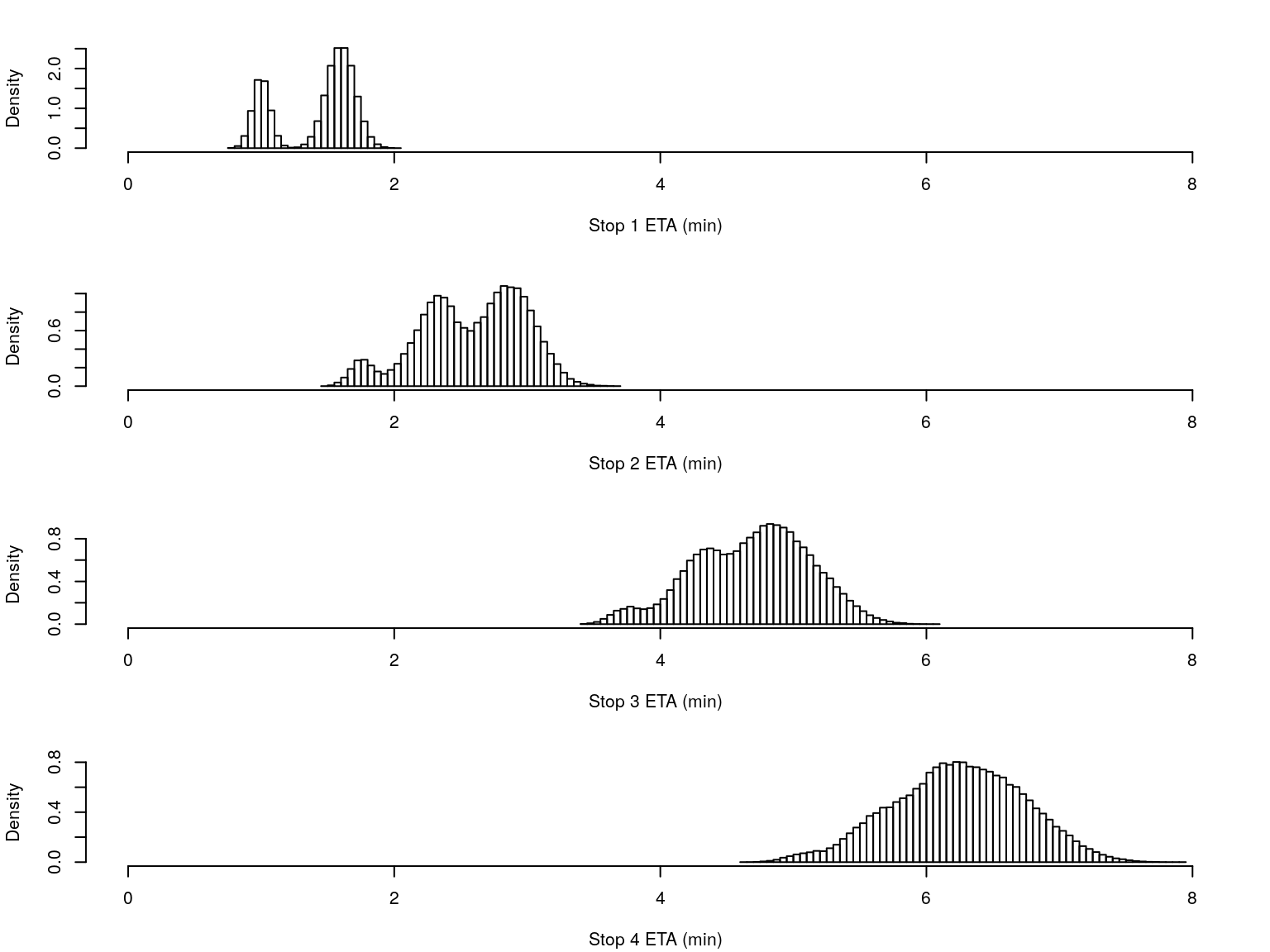# Part FourArrival time distrutions$$\downarrow$$ETAs

## Arrival time distribution

• we have a distribution
$p(A_j | \boldsymbol{x}_k, \Theta_c)$
• need to summarize it for the general public

## Point estimates

• expected
• easy to communicate
• infrastrature exists (real-time boards, etc)

## Point estimates ii

• assumes ETA is exact (it’s not)
• underestimate = frustration, hard to plan journey
• overestimate = shorter wait OR miss bus entirely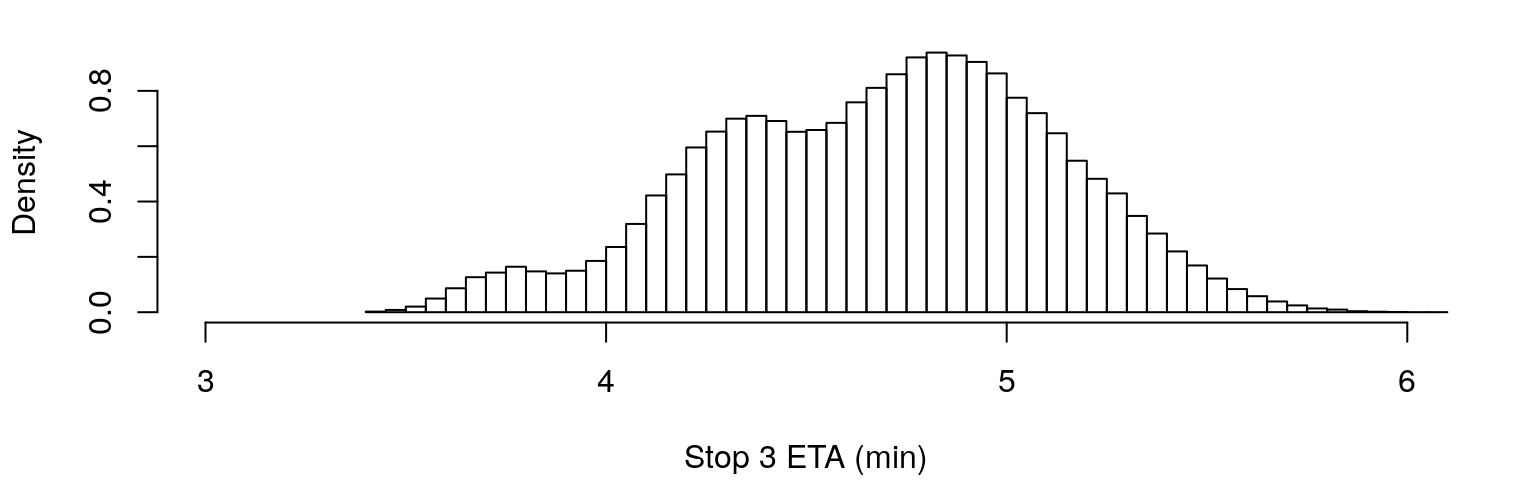• mean? median? some quantile?
• minimise $$p(A_j \notin [\hat A_j, \hat A_j+1)), A_j \in 0, 1, 2, \ldots$$
• … stronger penalty on overestimation?

### Point estimates iii

• desirable that $$A_j$$ decreases with time
• Kalman filter on arrival times? at least for > 10 mins

### Prediction intervals

• unfamiliar to the general public
• allows uncertainty to be expressed
• at least $$\ell_j$$ mins away, could be up to $$u_j$$ mins
• what values?
• quantiles of predictive distribution?
• min $$p(A_j \notin [\ell_j, u_j])$$ constrained by $$(u_j - \ell_j)^2$$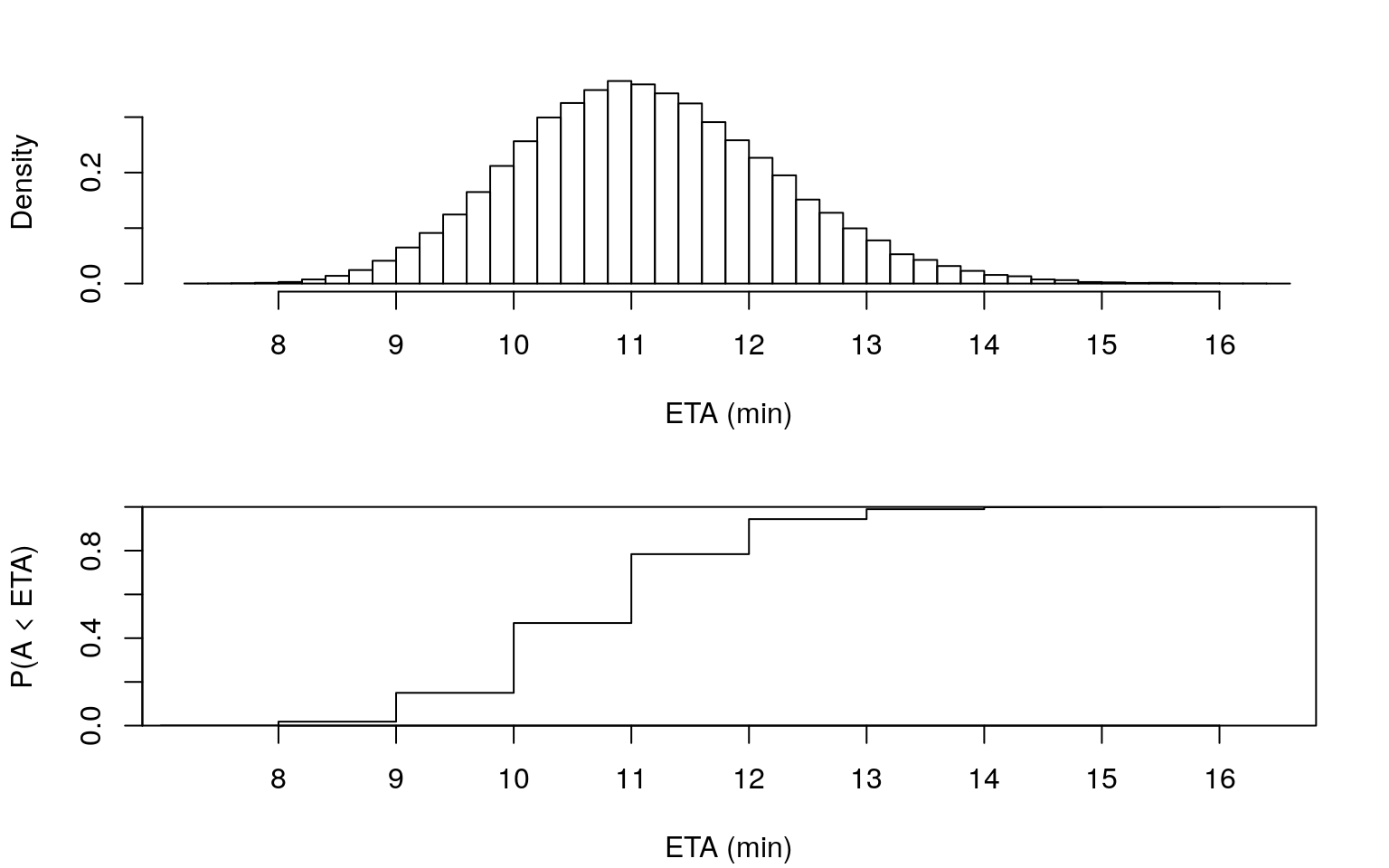Probability (%) bus arrives within x mins
7 8 9 10 11 12 13 14 15 16
0.05 1.8 15 46.9 78.4 94.4 99 99.874 99.991 100